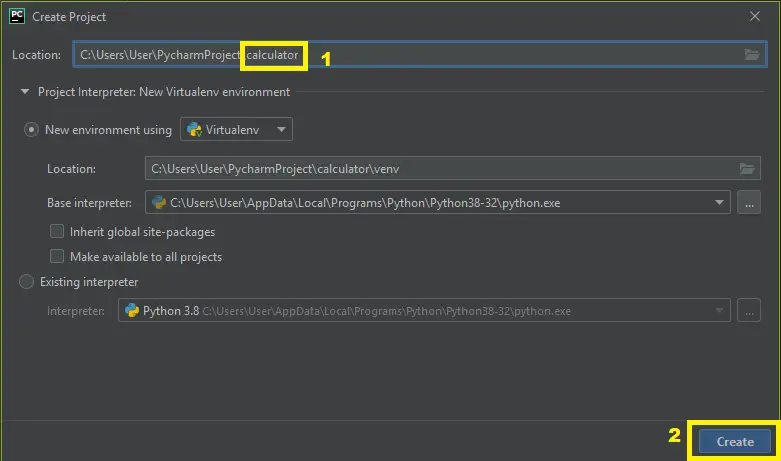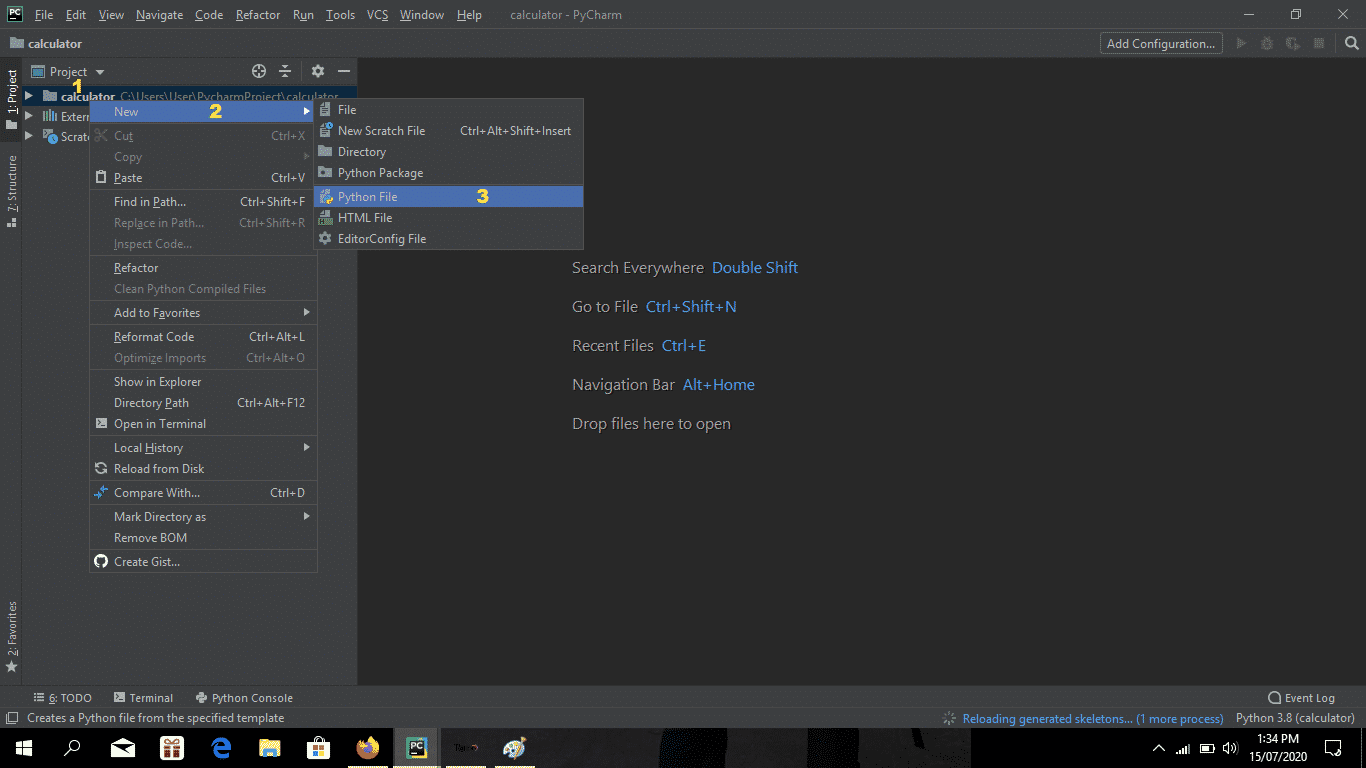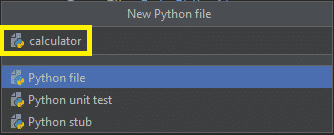# How To Make A Simple Calculator in Python? A Complete Guide

The Program For Calculator In Python is written in Python programming language, In this article I will teach you how to make a simple calculator in Python.

## Program For Calculator In Python: Project Information

Python has a design philosophy that emphasizes code readability. That’s why Python is very smooth to use mainly for novices who just started programming.

It is very smooth to research the syntax emphasizes clarity and it could reduce time consuming in developing.

## About The Project Calculator in Python

This Program was designed in Calculator In Python GUI or Graphical User Interface using Calculator In Python Tkinter.

To start creating the Program For Calculator In Python, make sure that you have PyCharm IDE installed on your computer.

## Recommendations

By the way, if you are new to Python programming and don’t know what Python IDE to use, I have here a list of Best Python IDE for Windows, Linux, and Mac OS that will suit you.

## Steps on How To Make A Calculator in Python with Example

Time needed: 6 minutes

How To Make A Calculator in Python with Example

• Step 1: Create a project name.

First, open PyCharm IDE and then create a “project name.” After creating a project name click the “create” button.• Step 2: Create a python file.

Second, after creating a project name, “right click” your project name and then click “new.” After that click the “python file“.• Step 3: Name your python file.

Third, after creating a Python file, Name your Python file after that click “enter“.• Step 4: The actual code.

Fourth is the actual coding on How To Create a Simple Calculator Program In Python and you are free to copy the given code below and download the full source code below.

## Calculator in Python Code Explanations

### 1. Import Module

The code given below is for importing the module.

``from tkinter import*``

This piece of code is importing the Tkinter module for the design of this project.

### 2. GUI

The code given below is for the design or GUI of this project.

``````def fCalc(src, side):
appObj = Frame(src, borderwidth=4, bd=2,bg = "#cccccc")
appObj.pack(side=side, expand=YES, fill=BOTH)
return appObj

def button(src, side, text, command=None):
appObj = Button(src, text=text, command=command)
appObj.pack(side=side, expand=YES, fill=BOTH)
return appObj

class app(Frame):
def __init__(self, root = Tk(), width=364, height=425):
Frame.__init__(self)
self.pack(expand=YES, fill=BOTH)
self.master.title("Simple Calculator")
screen_width = root.winfo_screenwidth()
screen_height = root.winfo_screenheight()
x = (screen_width/2) - (width/2)
y = (screen_height/2) - (height/2)
root.geometry('%dx%d+%d+%d' % (width, height, x, y))
display = StringVar()
Entry(self, relief= RIDGE,
textvariable=display, state=DISABLED, justify='right', bd=20, bg="silver").pack(side=TOP, expand=YES,
fill=BOTH)
clrChar = "Clear"
button(self, TOP, clrChar, lambda appObj=display, i=clrChar: appObj.set(''))

for btnNum in ("789/", "456*", "123-", "0.+"):

FunctionNum = fCalc(self, TOP)
for fEquals in btnNum:
button(FunctionNum, LEFT, fEquals,
lambda appObj=display, i=fEquals: appObj.set(appObj.get() + i))
EqualsButton = fCalc(self, TOP)

for fEquals in "=":
if fEquals == "=":
btnEquals = button(EqualsButton, LEFT, fEquals)
btnEquals.bind('<ButtonRelease-1>',
lambda e, s=self, appObj=display: s.result(appObj), "+")
else:
btnEquals = button(EqualsButton, LEFT, fEquals,
lambda appObj=display, s=" %s "%fEquals: appObj.set(appObj.get()+s))``````

In this module is the code for the design of this project.

### 3. Display Result

The code given below is for displaying the result.

``````    def result(self, display):
try:
display.set(eval(display.get()))
except:
display.set("UNDEFINED")``````

In this module which displays the result you’ve entered in the text field, with conditioning.

### 4. Complete Source Code

``````from tkinter import*

def fCalc(src, side):
appObj = Frame(src, borderwidth=4, bd=2,bg = "#cccccc")
appObj.pack(side=side, expand=YES, fill=BOTH)
return appObj

def button(src, side, text, command=None):
appObj = Button(src, text=text, command=command)
appObj.pack(side=side, expand=YES, fill=BOTH)
return appObj

class app(Frame):
def __init__(self, root = Tk(), width=364, height=425):
Frame.__init__(self)
self.pack(expand=YES, fill=BOTH)
self.master.title("Simple Calculator")
screen_width = root.winfo_screenwidth()
screen_height = root.winfo_screenheight()
x = (screen_width/2) - (width/2)
y = (screen_height/2) - (height/2)
root.geometry('%dx%d+%d+%d' % (width, height, x, y))
display = StringVar()
Entry(self, relief= RIDGE,
textvariable=display, state=DISABLED, justify='right', bd=20, bg="silver").pack(side=TOP, expand=YES,
fill=BOTH)
clrChar = "Clear"
button(self, TOP, clrChar, lambda appObj=display, i=clrChar: appObj.set(''))

for btnNum in ("789/", "456*", "123-", "0.+"):

FunctionNum = fCalc(self, TOP)
for fEquals in btnNum:
button(FunctionNum, LEFT, fEquals,
lambda appObj=display, i=fEquals: appObj.set(appObj.get() + i))
EqualsButton = fCalc(self, TOP)

for fEquals in "=":
if fEquals == "=":
btnEquals = button(EqualsButton, LEFT, fEquals)
btnEquals.bind('<ButtonRelease-1>',
lambda e, s=self, appObj=display: s.result(appObj), "+")
else:
btnEquals = button(EqualsButton, LEFT, fEquals,
lambda appObj=display, s=" %s "%fEquals: appObj.set(appObj.get()+s))

def result(self, display):
try:
display.set(eval(display.get()))
except:
display.set("UNDEFINED")

if __name__ == '__main__':
app().mainloop()

``````